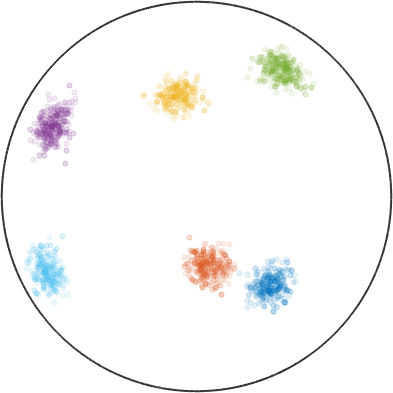Cluster demo edit page

This code demonstrates how the clustering algorithm can be used to assign vector3d and crystal directions to groups by proximity.

define an octohedral crystal symmetry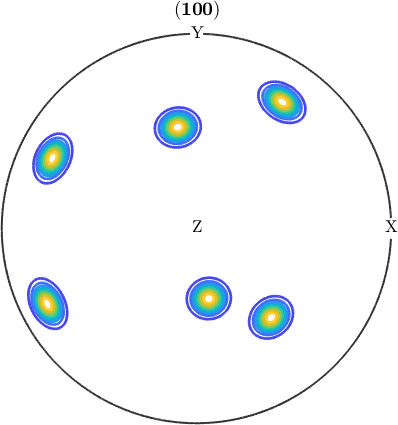assign each vector3d to one of twelve clusters, and calculate the vector3D located at the center of each cluster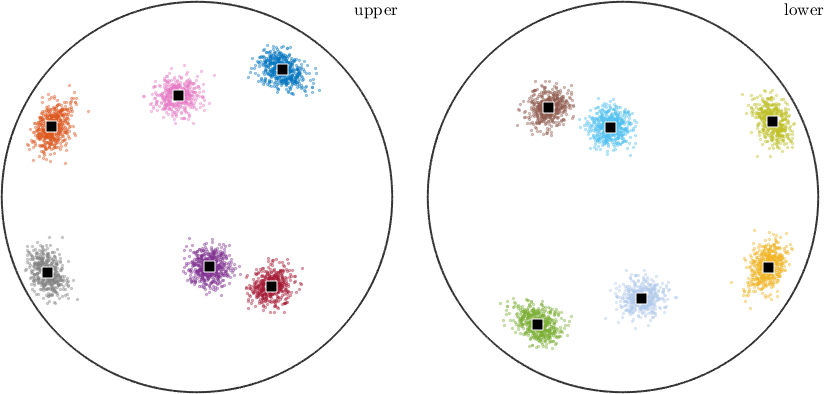Note that the upper and lower hemisphere plots are versions of each other, reflected horizontally plus vertically. This means that the underlying data has antipodal symmetry, contributing equally to both hemispheres. Let's include that in the cluster sorting.

repeat the calculation after changing all the vector3d to be antipodal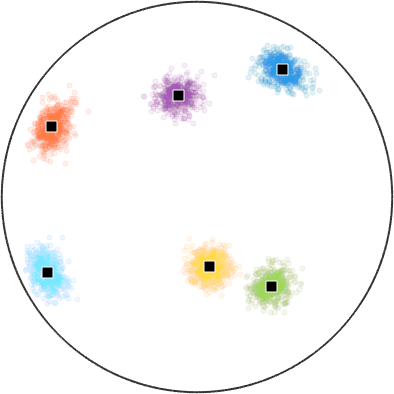pick a vector3d, and use that to convert the 10k random orientations previously generated into crystal directions.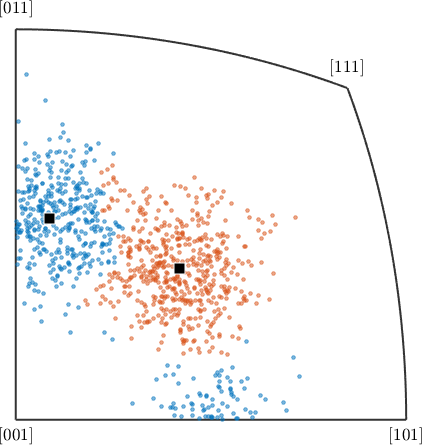just as we calculated clusters for vector3D and crystal directions, we're now going to do so for orientations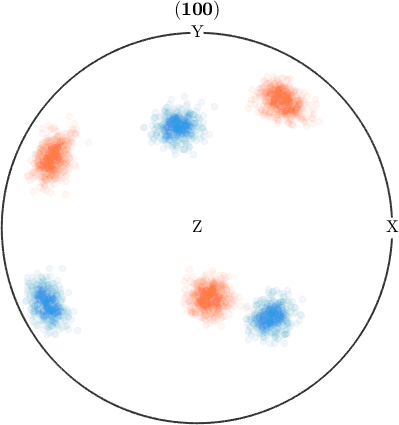If you have the statistics toolbox, you can make some calculations about the spread of points assigned to each cluster.